## How to Calculate and Solve for Steady State Diffusion | Diffusion in Alloying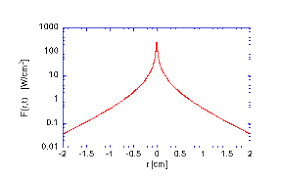The image above represents steady state diffusion.

To compute for steady state diffusion, three essential parameters are needed and these parameters are Diffusion Gradient (dC/dx), Cross-sectional Area (A) and Time (t).

The formula for calculating steady state diffusion:

J = dC/dx/At

Where:

A = Cross-sectional Area
t = Time

Given an example;
Find the steady state diffusion when the diffusion coefficient is 12, the cross-sectional area is 4 and time is 2.

This implies that;

dC/dx = Diffusion Gradient = 12
A = Cross-sectional Area = 4
t = Time = 2

J = dC/dx/At
J = 12/(4)(2)
J = 12/8
J = 1.5

Therefore, the steady state diffusion is 1.5mol m-2 s-1.

## How to Calculate and Solve for Diffusion Flux | Diffusion in Alloying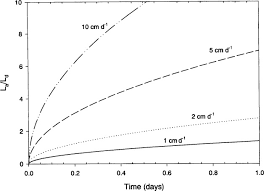The image above represents diffusion flux.

To compute for diffusion flux, three essential parameters are needed and these parameters are Mass Transfer (M), Cross-sectional Area (A) and Time (t).

The formula for calculating diffusion flux:

J = M/At

Where:

J = Diffusion Flux
M = Mass Transfer
A = Cross-sectional Area
t = Time

Given an example;
Find the diffusion flux when the mass transfer is 8, the cross-sectional area is 2 and the time is 6.

This implies that;

M = Mass Transfer = 8
A = Cross-sectional Area = 2
t = Time = 6

J = M/At
J = 8/(2)(6)
J = 8/12
J = 0.66

Therefore, the diffusion flux is 0.66 mol m-2 s-1.

## How to Calculate and Solve for Initial Grain Diameter | Deformation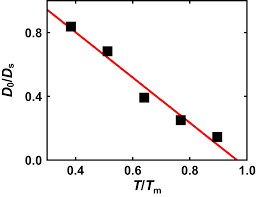The image above represents initial grain diameter.

To compute for initial grain diameter, four essential parameters are needed and these parameters are Average Grain Size Diameter (d), Time (t), Time Independent Constant (k) and Time Independent Constant (n).

The formula for calculating initial grain diameter:

do = (dn – kt)(1/n)

Where:

d = Average Grain Size Diameter
k, n = Time Independent Constants
t = Time

Given an example;
Find the initial grain diameter when the average grain size diameter is 2, the time is 3, the time independent constant is 6 and the time independent constant is 9.

This implies that;

d = Average Grain Size Diameter = 2
k = Time Independent Constant = 6
n = Time Independent Constant = 9
t = Time = 3

do = (dn – kt)(1/n)
do = (29 – (6)(3))(1/9)
do = (512 – (18))(1.992)
do = (494)(1.992)
do = 1.99

Therefore, the initial grain diameter is 1.99 mm.

## How to Calculate and Solve for Avrami Equation | Phase Transformation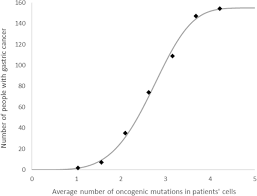The image above represents avrami equation.

To compute for avrami equation, three essential parameters are needed and these parameters are Time (t), Time Independent Constant for the Reaction (K) and Time Independent Constant for the Reaction (n).

The formula for calculating avrami equation:

y = 1 – exp(-Ktn)

Where:

y = Avrami Equation
K = Time Independent Constants for the Reaction
n = Time Independent Constants for the Reaction
t = Time

Let’s solve an example;
Find the avrami equation when the time independent constant for the reaction is 18, the time independent constant for the reaction is 10 and the time is 12.

This implies that;

K = Time Independent Constants for the Reaction = 18
n = Time Independent Constants for the Reaction = 10
t = Time = 12

y = 1 – exp(-Ktn)
y = 1 – exp(-(18)(12)10)
y = 1 – exp((-18)(61917364224))
y = 1 – exp(-1114512556032)
y = 1 – 0
y = 1

Therefore, the avrami equation is 1.

## How to Calculate and Solve for Corrosion Penetration Rate | Corrosion

The corrosion penetration rate is illustrated by the image below.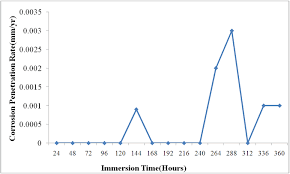To compute for corrosion penetration rate, five essential parameters are needed and these parameters are Constant (k), Weight Loss after Corrosion (W), Density of Corroding Media (ρ), Cross-sectional Area (A) and Time (t).

The formula for calculating corrosion penetration rate:

CPR = kW/ρAt

Where:

CPR = Corrosion Penetration Rate
k = Constant
W = Weight Loss after Corrosion
ρ = Density of Corroding Media
A = Cross-sectional Area
t = Time

Let’s solve an example;
Find the corrosion penetration rate when the constant is 2, the weight loss after corrosion is 4, the density of corroding media is 6, the cross-sectional area is 12 and time is 7.

This implies that;

k = Constant = 2
W = Weight Loss after Corrosion = 4
ρ = Density of Corroding Media = 6
A = Cross-sectional Area = 12
t = Time = 7

CPR = kW/ρAt
CPR = (2)(4)/(6)(12)(7)
CPR = 8/504
CPR = 0.0158

Therefore, the corrosion penetration rate is 0.0158.

## How to Calculate and Solve for Permeability Number | Refractories

The permeability number is illustrated by the image below.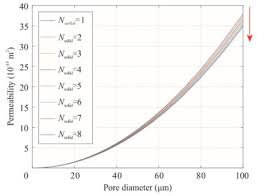To compute for the permeability number, five essential parameters are needed and these parameters are Volume of Air (V), Height of the Specimen (H), Cross-sectional Area (A), Air Pressure (P) and Time (t).

The formula for calculating permeability number:

PN = VH/APt

Where:

PN = Permeability Number
V = Volume of Air
H = Height of the Specimen
A = Cross-sectional Area
P = Air Pressure
t = Time

Let’s solve an example;
Find the permeability number when the volume of air is 2, the height of the specimen is 7, the cross-sectional area is 3, the air pressure is 9 and the time is 4.

This implies that;

V = Volume of Air = 2
H = Height of the Specimen = 7
A = Cross-sectional Area = 3
P = Air Pressure = 9
t = Time = 4

PN = VH/APt
PN = (2)(7)/(3)(9)(4)
PN = (14)/(108)
PN = 0.129

Therefore, the permeability number is 0.129.

## How to Calculate and Solve for Intensity of Radiation transmitted through Medium | Optical Properties

The intensity of radiation transmitted through medium is illustrated by the image below.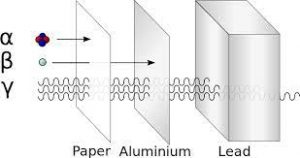To compute for intensity of radiation transmitted through medium, four essential parameters are needed and these parameters are

The formula for calculating intensity of radiation transmitted through medium:

IT = Io(1 – R)²e-βt

Where:

IT = Intensity of Radiation transmitted through Medium
Io = Intensity of Non-Reflected Incident Radiation
R = Reflectance
β = Absorption Coefficient
t = Time

Given an example;
Find the intensity of radiation transmitted through medium when the intensity of non-reflected incident radiaiton is 8, the reflectance is 2, the absorption coefficient is 4 and the time is 6.

This implies that;

Io = Intensity of Non-Reflected Incident Radiation = 8
R = Reflectance = 2
β = Absorption Coefficient = 4
t = Time = 6

IT = Io(1 – R)²e-βt
IT = 8(1 – 2)²e-(4)(6)
IT = 8(-1)²e-24
IT = 8(1)(3.775e-11)
IT = 3.020e-10

Therefore, the intensity of radiation transmittte through medium is 3.020e-10 W/sr.

## How to Calculate and Solve for Standard Permeability of Sand | Solidification of Metals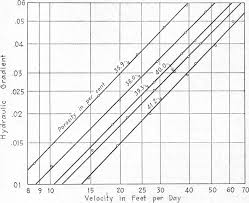The image above represents standard permeability of sand.

To compute for standard permeability of sand, five essential parameters are needed and these parameters are Volume of Area (V), Height of Sand Specimen (H), Pressure (Pr), Cross-Sectional Area of Sand Specimen (A) and Time (t).

The formula for calculating the standard permeability of sand:

P = VH / PrAt

Where:

P = Standard Permeability of Sand
V = Volume of Air
H = Height of Sand Specimen
Pr = Pressure
A = Cross-Sectional Area of Sand Specimen
t = Time

Let’s solve an example;
Find the standard permeability of sand when the volume of air is 6, the height of sand specimen is 3, the pressure is 8, the cross-sectional area of sand specimen is 5 and the time is 2.

This implies that;

V = Volume of Air = 6
H = Height of Sand Specimen = 3
Pr = Pressure = 8
A = Cross-Sectional Area of Sand Specimen = 5
t = Time = 2

P = VH / PrAt
P = 6 x 3 / 8 x 5 x 2
P = 18 / 80
P = 0.225

Therefore, the standard permeability of sand is 0.225.

Calculating the Volume of Air when the Standard Permeability of Sand, the Height of Sand Specimen, the Pressure, the Cross-Sectional Area of Sand Specimen and the Time is Given.

V = P x PrAt / H

Where:

V = Volume of Air
P = Standard Permeability of Sand
H = Height of Sand Specimen
Pr = Pressure
A = Cross-Sectional Area of Sand Specimen
t = Time

Let’s solve an example;
Find the volume of air when the standard permeability of sand is 10, the height of sand specimen is 2, the pressure is 4, the cross-sectional area of sand specimen is 8 and the time is 1.

This implies that;

P = Standard Permeability of Sand = 10
H = Height of Sand Specimen = 2
Pr = Pressure = 4
A = Cross-Sectional Area of Sand Specimen = 8
t = Time = 1

V = P x PrAt / H
V = 10 x (4)(8)(1) / 2
V = 320 / 2
V = 160

Therefore, the volume of air is 160.

## How to Calculate and Solve for Thickness of Solidifying Metals | Solidification of Metals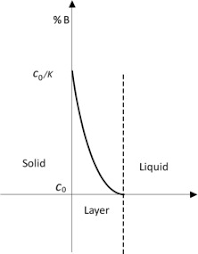The image above represents thickness of solidifying metals.

To compute for thickness of solidifying metals, six essential parameters are needed and these parameters are Melting Temperature of Metal (Tm), Initial Mould Temperature (To), Heat Diffusivity (α), Time (t), Density (ρ’) and Latent Heat of Fusion (Hf).

The formula for calculating thickness of solidifying metals:

M = 2(Tm – To)√(α)√(t) / √(π)ρ’Hf

Where:

M = Thickness of Solidifying Metal
Tm = Melting Temperature of Metal
To = Initial Mould Temperature
α = Heat Diffusivity
t = Time
ρ = Density
Hf = Latent Heat of Fusion

Let’s solve an example;
Find the thickness of solidifying metal when the melting temperature of metal is 4, the initial mould temperature is 8, the heat diffusivity is 2, the time is 6, the density is 3 and the latent heat of fusion is 7.

This implies that;

Tm = Melting Temperature of Metal = 4
To = Initial Mould Temperature = 8
α = Heat Diffusivity = 2
t = Time = 6
ρ = Density = 3
Hf = Latent Heat of Fusion = 7

M = 2(Tm – To)√(α)√(t) / √(π)ρ’Hf
M = 2(4 – 8)√(2)√(6) / √(π)(3)(7)
M = 2(-4)(1.414)(2.449) / (1.772)(3)(7)
M = -27.712 / 37.22
M = -0.744

Therefore, the thickness of solidifying metal is -0.744 m.

## How to Calculate and Solve for Total Heat Loss in Furnace | Fuel and Furnaces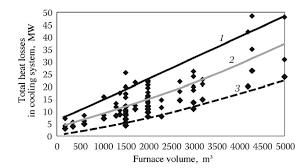The image above represents total heat loss in furnace.

To compute for total heat loss in furnace, four essential parameters are needed and these parameters are Absolute Temperature (Kelvin) (T), Factor of Total Radiation (a), Area of Opening (A) and Time (Hours) (H).

The formula for calculating total heat loss in furnace:

Q = 4.88(T/100)4 x a x AH

Where:

Q = Total Heat Loss in Furnace
T = Absolute Temperature (Kelvin)
a = Factor of Total Radiation
A = Area of Opening
H = Time (Hours)

Let’s solve an example;
Find the total heat loss in furnace when the absolute temperature is 22, the factor of total radiation is 14, the area of opening is 10 and the time is 18.

This implies that;

T = Absolute Temperature (Kelvin) = 22
a = Factor of Total Radiation = 14
A = Area of Opening = 10
H = Time (Hours) = 18

Q = 4.88(T/100)4 x a x AH
Q = 4.88(22/100)4 x 14 x (10)(18)
Q = 4.88(0.22)4 x 14 x 180
Q = 4.88(0.00234256) x 2520
Q = 28.807

Therefore, the total heat loss in furnace is 28.807 J/Kg K.